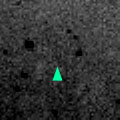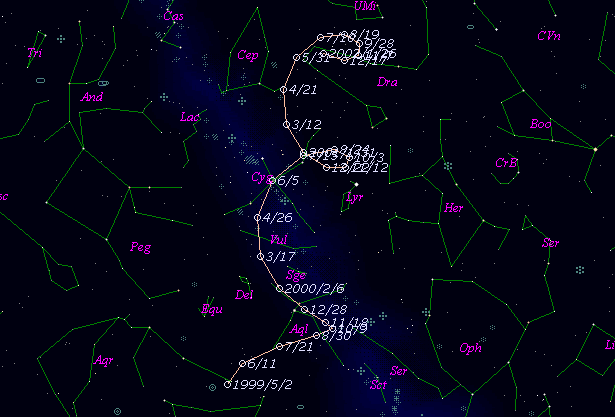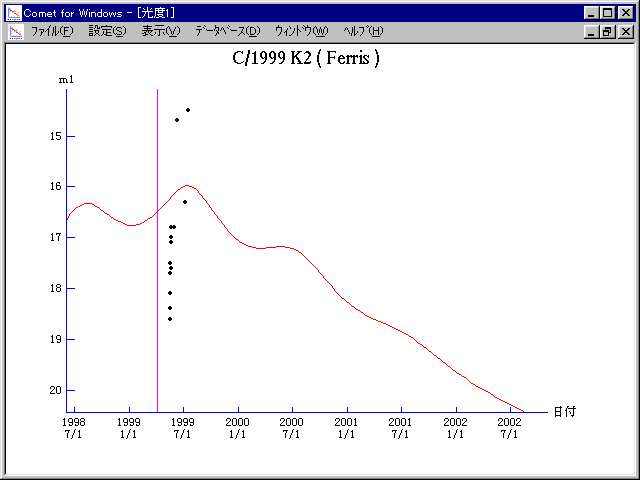# \$B%U%'%j%9WB@1(B

C/1999 K2 ( Ferris )###\$B%W%m%U%#!<%k(B

 \$BH/8+F|(B 1999\$BG/(B 5\$B7n(B19\$BF|(B \$BH/8+8wEY(B 18.1\$BEy(B \$BH/8+ William D. Ferris (LONEOS)

###\$B###\$B50F;MWAG(B

```   The following improved orbital elements by Kenji Muraoka, are
from 90 observations  1999 May 19  to  Aug. 22, perturbations
by 9 Planets, Moon and 5 minor planets were taken into account.
The mean residual is +/- 0.69 arc seconds.

Epoch  =  1999 Apr. 12.0  TT       JDT = 2451280.5
T  =  1999 Apr. 10.48873       +/- 0.07044 (m.e.) TT
Peri. =    4.56506                +/- 0.00608
Node  =  300.31861                +/- 0.00219   (2000.0)
Incl. =   82.19189                +/- 0.00560
q  =    5.2903914              +/- 0.0001858 AU
e  =    0.9659754              +/- 0.0001730
1/a  =   +0.0064314              +/- 0.0000327 1/AU
( P  =   1939 years )
orig. 1/a  =   +0.006869
fut.  1/a  =   +0.007056
```

###\$B@1?^(B###\$B8wEYJQ2=(B

```        m1 = 5.5 + 5 log\$B&\$(B + 10.0 log r
```##### \$B50F;MWAG\$OB<2,7r<#;a\$N7W;;\$K\$h\$k\$b\$N\$G\$9!#(B \$B@1?^\$O(B StellaNavigator Ver.2.0 for Windows (\$B%"%9%H%m%"!<%D(B \$BJTCx(B / \$B%"%9%-!<=PHG6I4)(B) \$B\$G:n@.\$7\$?\$b\$N\$G\$9!#(B \$B8wEY%0%i%U\$O(BComet for Windows\$B\$G:n@.\$7\$?\$b\$N\$G\$9!#(B